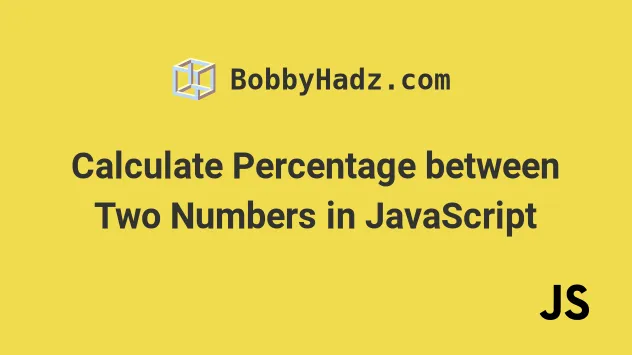# Calculate Percentage between Two Numbers in JavaScriptLast updated: Jul 25, 2022Photo from Unsplash

## Calculate Percentage between Two Numbers#

To calculate the percentage between two numbers, divide one number by the other and multiply the result by 100, e.g. `(30 / 75) * 100`. This shows what percent the first number is of the second, in the example - `30` is `40%` of `75`.

index.js
```Copied!```function isWhatPercentOf(numA, numB) {
return (numA / numB) * 100;
}

// 👇️ `30` is 40% of `75`
console.log(isWhatPercentOf(30, 75)); // 👉️ 40

// 👇️ `20` is 26.666% of `75`
console.log(isWhatPercentOf(20, 75)); // 👉️ 26.666666...

// ✅ Round to 2 decimals
console.log(isWhatPercentOf(20, 75).toFixed(2)); // 👉️ "26.67"

// ✅ Get percentage Increase / Decrease
function getPercentageIncrease(numA, numB) {
return ((numA - numB) / numB) * 100;
}

// 👇️ `50` is 66.66% increase from `30`
console.log(getPercentageIncrease(50, 30)); // 👉️ 66.666

// 👇️ `50` is 50% decrease from `100`
console.log(getPercentageIncrease(50, 100)); // 👉️ -50
``````

The first function we declared takes 2 numbers are returns what percent numberA is of numberB.

For example, `25 / 50 * 100` shows that `25` is `50%` of `50`.

index.js
```Copied!```// 👇️ `25` is 50% of `50`
console.log((25 / 50) * 100);
``````
When calculating percentages between two numbers, you might need to round to a specific number of digits after the decimal.

You can use the Number.toFixed method to achieve this.

index.js
```Copied!```const percentage = (20 / 75) * 100;
console.log(percentage); // 👇️ 26.666666666...

// 👇️ 2 decimals
const fixed = percentage.toFixed(2);
console.log(fixed); // 👉️ "26.67"
``````
The `toFixed` method formats the number to the provided number of digits after the decimal and rounds the number if necessary.

Note that the `toFixed` method returns a string, not a number.

If the number doesn't have any decimal places, it gets padded with zeros.

index.js
```Copied!```const percentage = (50 / 100) * 100;
console.log(percentage); // 👇️ 50

// 👇️ 2 decimals
const fixed = percentage.toFixed(2);
console.log(fixed); // 👉️ "50.00"
``````

Our second function shows how to get the percentage increase or decrease between two numbers.

index.js
```Copied!```function getPercentageIncrease(numA, numB) {
return ((numA - numB) / numB) * 100;
}

// 👇️ `50` is 66.66% increase from `30`
console.log(getPercentageIncrease(50, 30)); // 👉️ 66.666

// 👇️ `50` is 50% decrease from `100`
console.log(getPercentageIncrease(50, 100)); // 👉️ -50
``````

The first example shows that the percentage increase from `30` to `50` is `66.6666...%`.

And the second example shows that the percentage increase from `100` to `50` is `-50%`.